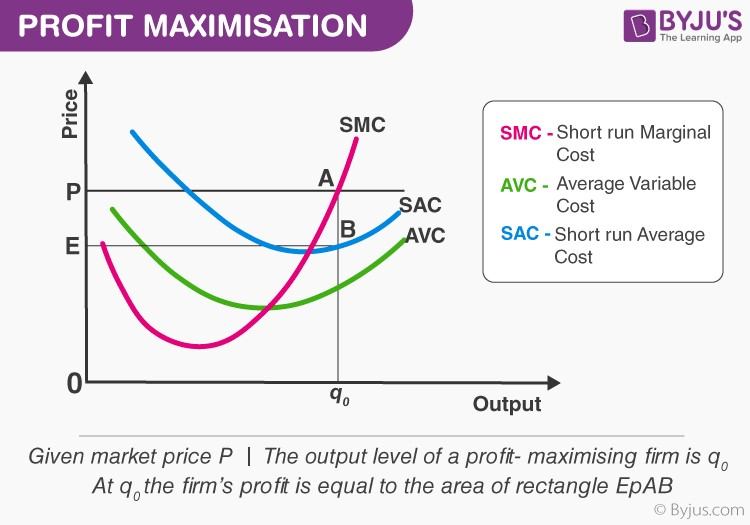# The Profit Maximisation Problem: Graphical Representation

The normal rule of profit maximisation is that the enterprise maximises the profit by manufacturing that amount of output, where the marginal revenue equals the marginal cost. The profit maximisation issue can also be approached from the input outlook.Now, let us graphically depict an enterprises profit market cost price be p. Equalising the market cost price with the (short-run) marginal cost, we procure the output degree q0. At q0, notice that SMC slope is higher and p surpasses AVC.

Since the three conditions explained in the earlier sections are contented at q0, we keep that the profit-maximising output degree of the enterprise is q0

What happens at q0? The total revenue of the enterprise at q0 is the area of rectangle OpAq0 (the product of cost price and quantity), while the total cost at q0 is the area of rectangle OEBq0 (the product of short-run average cost and quantity). So, at q0, the enterprise earns a profit equal to the area of the rectangle EpAB.

This was a detailed and elucidated information about the concept of The Profit Maximisation Problem: Graphical Representation. To learn more, stay tuned to BYJUS.